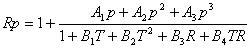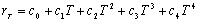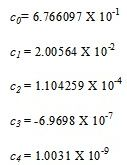# Practical Salinity Scale – 1978

The Practical Salinity Scale 1978 (PSS-78) has been considered by the Joint Panel on Oceanographic Tables and Standards and recommended by all oceanographic organizations as the scale in which to report future salinity data.

Definition of the practical salinity:

Practical salinity, symbol S, of a sample of seawater, is defined in terms of the ratio K15 of the electrical conductivity of the seawater sample at the temperature of 15°C and the pressure of one standard atmosphere, to that of a potassium chloride (KCl) solution, in which the mass fraction of KCl is 32.4356E-3, at the same temperature and pressure. The K15 value exactly equal to 1 corresponds, by definition, to a practical salinity exactly equal to 35, The practical salinity is defined in terms of ratio K15 by the following equation:formulated and adopted by the UNESCO/ICES/SCOR/IAPSO Joint Panel on Oceanographic tables and Standards, Sidney, BC, Canada, 1-5 September 1980. PSS-78 is based on an equation relating salinity to the ratio of the electrical conductivity of seawater at 15°C to that of a standard potassium chloride solution (KCl). It has already been pointed out that a “conductivity ratio” defined salinity scale is better than a “chlorinity” scale for density determination; and added to this is the study of Farland showing that in the hands of average observers, titration is a less precise than is conductivity measurement. In order to eliminate the ambiguity exhibited by (1) and (2) under conditions of ionic ratio variation, the Practical Salinity Scale 1978 breaks the existing chlorinity-salinity tie in favor of a definite salinity-conductivity ratio relationship; all waters of the same conductivity ratio then have the same salinity. A standard seawater of 35 has by definition a conductivity ratio of unity at 15°C with a KCl solution containing a mass of 32.4356 g KCl in a mass of 1 kg of solution. In practice Merk “Suprapur” KCl has been found to be of adequate purity being consistent within a bath and between batches. It is worth noting that the major impurity is NaCl, and at the level of interest the molal conductivities of the two salts are sufficiently near to minimize the effect of the impurity. From measurements on weight diluted (with pure water) or weight evaporated North Atlantic surface water, the following relationship was established:where:

a0 = 0.008                 b0 = 0.0005
a1 =-0.1692               b1 = -0.0056
a2 = 25.3851              b2 = -0.0066
a3 = 14.0941              b3 = -0.0375
a4 = -7.0261               b4 = 0.0636
a5 = 2.7081                b5 = -0.0144

k = 0.0162              -2°C < T < 35°C

In all cases temperatures are measured according to the International Practical Temperature Scale (1968).
The values of coefficients in PSS-78 are based on experiments carried out on existing standard seawaters that were diluted and evaporated by weight. This ensures the conservatism of a salinity so defined and its local reproducibility. The standard deviation was 0.0013 in salinity. This equation is used for calculation of the practical salinity measured only by laboratory salinometers, where Rt is a measured value.
A supplementary equation was also established for converting conductivity ratios measured at pressure greater then one atmosphere (i.e. for CTD-measurements).
Given in situ measurements of conductivity ratio:

C(35,1568,0) = 42.914 mS/cm = 4.2914 S/m

T68 = 1.00024×T90
T68 – temperature IPTS-68, °C
T90 – temperature ITS-90,ºCwhere:where:
A=  2.070×10-5 B1 = 3.426×10-2
A= -6.370×10-10 B2 = 4.464×10-4
A=  3.989×10-15 B= 4.215×10-1
B= -3.107×10-3

The standard deviation was 1.3 ppm in salinity. Given R, T, and p, a may be computed and the factor rt, the temperature coefficient of standard seawater, may be obtained from the polynomial equation:where:These equations enable the practical salinity S in a range 2 to 42 to be calculated from conductivity C (mS/cm or S/m) measured at temperature T (ºC) and hydrostatic pressure p(dBar) and is valid in the temperature range -2°C to +35°C and hydrostatic pressure 0-10000 dBar.

Practical salinity should be expressed by dimensionless number only and should be written as, e.g. S = 35.034.